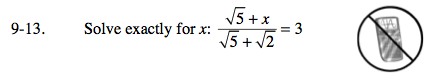### Home > CAAC > Chapter 16 > Lesson 16.9.1.1 > Problem9-13

9-13.$\text{Multiply both sides by }\sqrt{5} + \sqrt{2}.$

$\sqrt{5} + \textit{x} = 3(\sqrt{5} + \sqrt{2})$

Distribute.

$\sqrt{5} + \textit{x} = 3\sqrt{5} + 3\sqrt{2}$

$\text{Subtract }\sqrt{5}\text{ from both sides.}$

$x = 3\sqrt{5} + 3\sqrt{2} - \sqrt{5}$

Simplify.

$x = 2\sqrt{5} + 3\sqrt{2}$